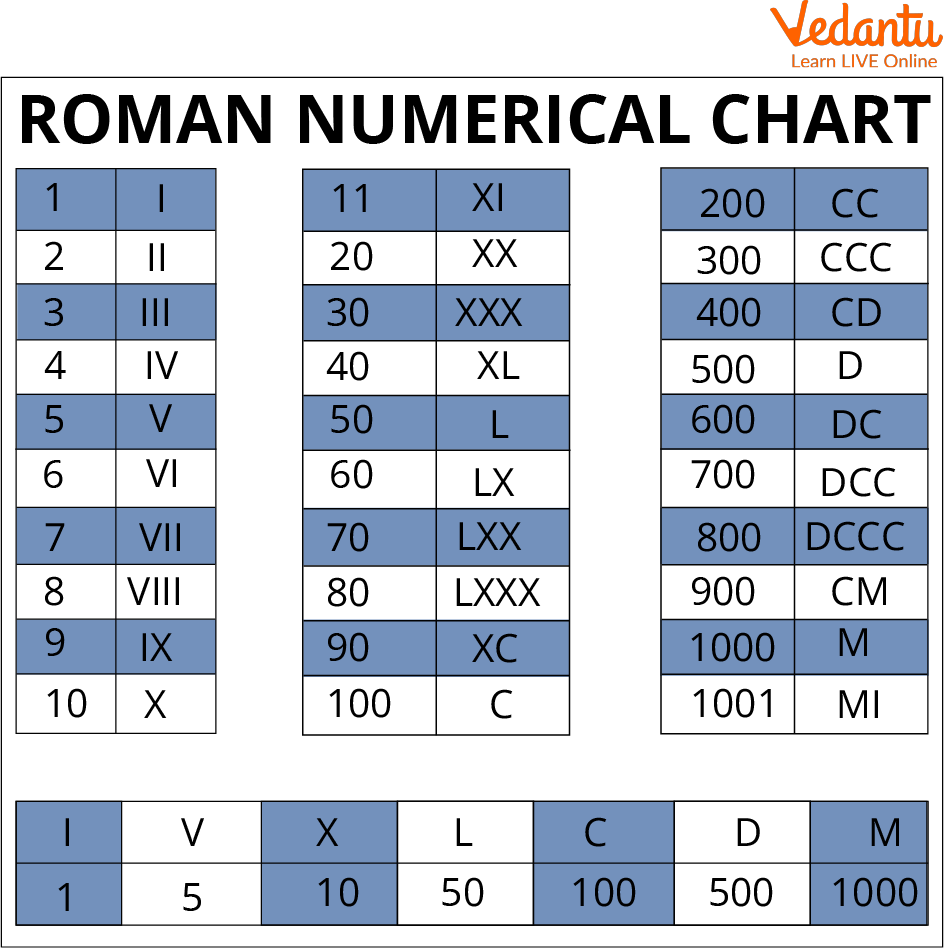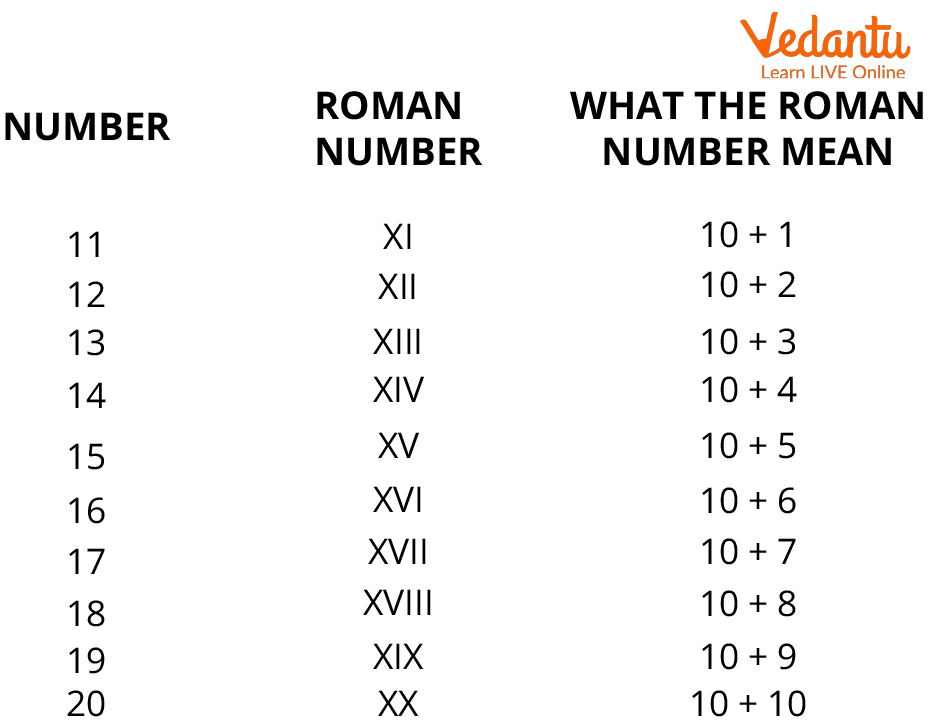Courses
Courses for Kids
Free study material
Free LIVE classes
More

# Roman Numerals to NumbersLIVE
Join Vedantu’s FREE Mastercalss

## Introduction to Roman Numerals

Roman numerals are a system of numeration that dates back to ancient Rome and were widely used to write numbers throughout Europe, until the late Middle Ages. Letters from the Latin alphabet are combined to produce the Roman Number System. Modern symbols consist of seven symbols and fixed integer values.

In modern times, roman numerals are used in many things. You can see roman numerals used in clocks to represent time, used outside or at the door of a room in school, office, college to represent room number, etc. You will learn about roman numerals to numbers and conversions related to it in this article.

## How to Write Roman Numbers?

Roman numbers are written by using basic symbols of roman numerals like

 Symbol I V X L C D M Value 1 5 10 50 100 500 1000

We only need the first 4 symbols to read and write integers up to 50.

Which are I V X L.

A single line, or "I," stood for a single entity, whereas "V" stood for five fingers, notably the V-shaped formation of the thumb and forefingers and "X" stands for two hands.

As a result, the Romans utilised a variety of symbol combinations to write numbers using addition and subtraction.

1. Symbol “I” has the value 1, and it will not be repeated more than three times.

2. Symbol “V” has the value 5, and it will not be repeated.

3. Symbol “X” has the value 10, and yes, to the max, it can be repeated three times maximum.

4. Symbol “L” has the value of 50, and it is not repeated.

5. Symbol “C” has the value 100, and it is repeated three times.

6. Symbol “M” has the value 1000, and it is repeated max three times.

So, above are some rules that we have to consider while explaining numerals into Roman Numerals.Chart showing Roman Numerals

## Convert Roman Numerals to Numbers

In Roman numerals, letters are used in place of numbers. Now, you must be familiar with the given seven letters: I = 1, V = 5, X = 10, L = 50, C = 100, D = 500, and M = 1000.

To create numbers, you combine the letters. Here are a few basic illustrations:

1) III = 3

Three are added together to equal three 1s because 1 + 1 + 1 is three.

2) XVI = 16

10 + 5 + 1 = 16

Roman numerals to numbers or numbers to the Roman numeral and the meaning of the Roman numeral is depicted by the following example of numbers from 11 to 20.Meaning of Roman Numeral

## Convert Roman Number to Integer

An integer is a number with no decimal or fractional part, from the set of negative and positive numbers, including zero. Examples are 56, 3, 0, and -4. Except in a few rare circumstances where the left character is less than the right character, Roman numerals are typically written from highest to lowest from left to right.

For instance, "IV" rather than "IIII" stands for 4. Subtract the left character value from the right character value in these circumstances. IV will be represented by 5-1 = 4, and IX by 10-1 = 9.

### Cases are listed below:

• I can come before V or X and stand for a one-per cent subtraction, so IV (5 - 1) = 4 and IX (10 - 1) = 9, respectively.

• X can come before L or C to stand for subtracting 10, making XL (50-10) equal to 40 and XC (100-10) equal to 90.

• C before a D or M stands for a hundred that has been subtracted, so CD (500-100) = 400 and CM (1000-100) = 900.

## Solved Examples

1. Convert these numbers to roman numerals.

1. 36

2. 42

3. 101

4. 51

Ans: (a) 36 = XXXVI

(b) 42 = XLII

(c) 101 = CI

(d) 51 = LI

2. Convert XLIV into an integer.

Ans: XLIV = 45-1 = 44

## Practice Questions

1. Convert the Roman numeral to numbers.

1. LIV

2. XLV

Ans: (a) 54, (b) 45

2. Convert the numbers to Roman numerals.

1. 100

2. 500

Ans: (a) C, (b) D

## Summary

Roman numerals are a type of number system that was used in ancient Rome. They are made up of a combination of Latin alphabetic letters. Modern symbols consist of seven symbols and fixed integer values. It is symbolised by a variety of symbols, including I, V, and X. In essence, it is a base ten or decimal number system. Conversion of roman numbers is discussed in this article like roman numbers to integers, roman numerals to numbers.

Roman numerals have never been subject to a formal, approved standard. In ancient Rome, usage was extremely diverse and, during the Middle Ages, completely disorganised.

Last updated date: 29th Sep 2023
Total views: 120.6k
Views today: 1.20k

## FAQs on Roman Numerals to Numbers

1. How do we write 26 in roman numerals?

To write 26 into roman numerals, expanding it, we get 26 = 10 + 10 + 5 + 1. So, it can be represented as XXVI.

2. Who was the roman numeral’s creator?

The Romans created a system of numerals to represent numbers around 500 BCE. For expressing numbers, the Roman numeral system was developed. When the Romans conquered a significant chunk of the world that was then known to them, Roman numerals became the norm for centuries to display numbers in Europe.

3. Which roman numeral cannot be repeated more than once?

Only one instance of the letters V, L, and D are ever used. A symbol should never be used more than three times in a row. Romans also permitted subtraction when a smaller number came before a larger one in order to keep numbers from growing too long and complicated.